American Journal of Aerospace Engineering
Volume 3, Issue 1, February 2016, Pages: 1-5

Measurement of the Liquid Level in the Fuel Tank of Rocket

N. I. Klyuev

Department of Mathematical Modeling in Mechanics, Samara State Aerospace University, Samara, Russia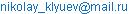N. I. Klyuev. Measurement of the Liquid Level in the Fuel Tank of Rocket. American Journal of Aerospace Engineering. Vol. 3, No. 1, 2016, pp. 1-5. doi: 10.11648/j.ajae.20160301.11

Received: February 17, 2016; Accepted: March 19, 2016; Published: April 6, 2016

Abstract: In this paper we consider the problem of mathematical modeling of fluid flow in the vertical channel of fuel management system. The system has a vertical measuring channel with sensors inside the channel for fixing the free surface level of fluid in the channel. By lowering the level of fuel in the tank fuel level decreases in the channel. When the level of fuel in the channel reaches the sensor, the sensor activation occurs. Thus, the level of fuel in the channel determining fuel level in the tank. Fluid flow in the vertical measuring channel describes by non-stationary equation of motion of parabolic type. For dynamic modeling used a viscous incompressible fluid. The fluid flow is called non-stationary parabolic equation of motion for the cylindrical channel. We got an approximate solution of the problem by averaging the terms of the equation of motion for the channel radius. The solution of the differential equation is satisfied in the package Mathcad applications. Graphs of displacement and velocity of the free surface of fuel in the measuring channel are represented over time. Measurement error of liquid level in the fuel tank has been determined. It is proposed engineering solution to eliminate error of the fuel level measurement.

Keywords: Viscous Liquid, Unsteady Flow, Liquid Level, Cylindrical Channel, Vibration, Measuring Error

Contents

1. Introduction

A fuel of the oxidizer tank and combustible tank flows into the combustion chamber of the rocket engine. Simultaneous supply of fuel at a predetermined proportion, ensures efficient operation of a rocket engine. Synchronous fuel delivery, at a predetermined proportion, enables efficient operation of the rocket engine. Efficient operation is dependent on accurate measurement of the fuel level in the tank. For this purpose, the fuel tank has a fuel management system. The system is a vertical measuring channel with sensors inside the channel for fixing the free surface level of fluid in the channel (Figure 1).

Vertical channel and fuel tank are communicating vessels. By lowering the level of fuel in the tank, fuel level decreases in the channel. When the level of fuel in the channel reaches the sensor, the sensor activation occurs. The signal goes to the fuel management system. As a result, the fuel consumption varies. Thus, the level of fuel in the channel determins fuel level in the tank. The problem is that the free surface of the fuel do not coincide in the channel and the tank. Error in measuring the level of fuel leads to inefficient fuel consumption. As a result, the rocket engine is not operating optimally and tanks have the "extra" amount of fuel.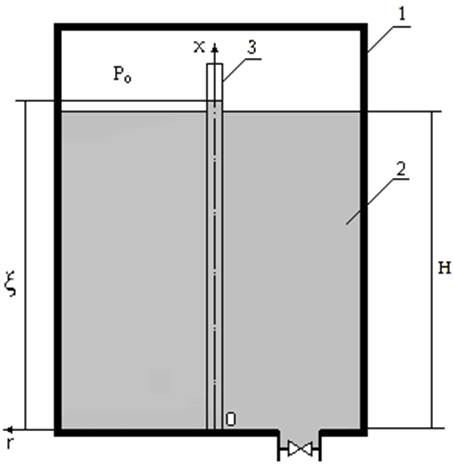Figure 1. Diagram of a fuel tank. 1- tank, 2- fuel, 3- measurement channel,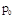- gas pressure,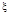- liquid level in the channel,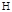- liquid level in the tank,- coordinate axis.

We consider the problem of mathematical modeling of fluid flow in the vertical channel of fuel level management system of rocket. Fluid flow in the vertical measuring channel describes by non-stationary equation of motion of parabolic type. Unsteady motion equation was solved by many researchers. Problems discussed in [1-3] can be called a classic. The article  considers the laminar fluid flow in a cylindrical channel from standstill. The pulsating motion is presented in . The results of calculation of a periodic motion are compared to the experimental data. The equations of motion are solved by operational calculus.

In  it is shown that in practical usage of rocket engines, the ratio of oxidizer and combustible is broken. What leads to a greater or lesser throttle opening. The paper  presents a non-linear mathematical model to simulate the pulsating flow. The governing equations are numerically solved by a composite scheme incorporating the two-step Lax-Wendroff method with the trapezoidal integration version of the method of characteristics at the pipe boundaries. The validity of the mathematical model and the methodology was verified by comparing the analytical predictions with experimental results.

Work  presents unsteady flow of a non-Newtonian fluid due to instantaneous valve closure. the appropriate governing equations are solved by a numerical approach. A fourth-order Runge-Kutta scheme is used for the time integration, and the central difference scheme is employed for the spatial derivatives discretization. To verify the proposed mathematical model and numerical solution, a comparison with corresponding experimental results from the literature are made.

In work  presents the method of characteristics (MOC) for simulation of unsteady flow in a pipeline. However, the relatively complex method of implicit (MOI) provides the advantages of unconditional convergence and mutual independence between time and space mesh parameters. This study combines the MOC and MOI to simulate pipeline unsteady flow and hydropower transient processes.

The paper  presents results of an investigation of the response of an incompressible fluid in a circular micro pipe to a sudden time-independent pressure drop. Solutions of the problem were obtained analytically using the Laplace transform technique and numerically using the lattice Boltzmann method.

The paper  presents steady and unsteady flows in a mildly curved pipe for a wide range of Reynolds numbers are examined with direct numerical simulation. In article  fully developed pulsed flow of an incompressible Newtonian fluid (crude oil) through a pipe line has been modeled and analyzed using the finite element method and compared with results based on an analytical solution. The flow is generated by a periodic pressure gradient superimposed on a constant Poiseuille flow. The results show good agreement between analytical and numerical solutions based on finite element method for the Newtonian fluid under unsteady regimes.

In article  the dynamic interaction between the pipe and unsteady flows is analyzed based on experiments and numerical models. The paper  presents a method of characteristics for solving one-dimensional model of fluid flow in pipe networks.

The goal of this research is to develop a mathematical model of the liquid flow in the vertical measuring channel of the fuel level measurement system of rocket.

2. The Mathematical Formulation of the Problem

At the initial time,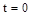, the fuel level in the tank and in the channel is the same (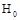). The free upper end of the cylindrical channel is located above the fuel level in the tank, so the flow of fuel from the tank into the channel at this location possible. The lower base of the cylindrical channel coincides with the bottom of the tank, and the fuel is communicated freely between the tank and the channel. Above the free surface of fuel (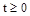) in the tank and the channel maintains a constant pressure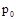(boost pressure).

The fuel level in the tank varies by the law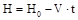.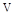- lowering speed of fuel in the tank. It is known that the liquid levels in the tank and the channel do not coincide. It is necessary to determine the level of liquid in the cylindrical channel at an arbitrary time. We introduce a coordinate system, the beginning of which was placed in the center of the lower base of the cylindrical channel.

As a model for the flow is used non-stationary motion of a viscous incompressible fluid in a cylindrical channel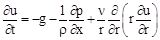(1)

boundary conditions of the problem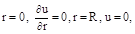the initial condition of the problem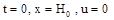,

where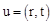- velocity of the liquid in the channel,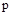- pressure,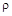- density,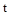- time,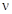- kinematic viscosity,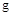- acceleration of gravity.

3. Method for Solving Problem

The average speed of fuel in the cylindrical channel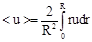. Multiplying the left and right sides of the equation (1) by r. We write down the individual terms of the equation of motion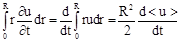,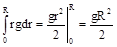,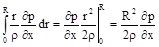,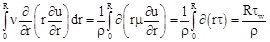(2)

where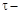friction,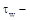wall friction,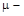dynamic viscosity,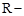radius of the cylindrical channel.

We use formulas (2) and write the equation (1) in the form (slanting braces at medium speed hereinafter omitted)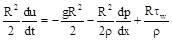or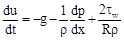(3)

Select in the cylindrical channel the volume of liquid by two cross sections at a distance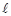. Write down the balance of pressure and friction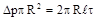.

We obtain the following expression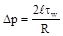(4)

where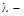drag coefficient of friction.

Then, we use the equation of Darcy-Weisbach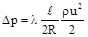,

uniting the equation of Darcy-Weisbach and equation (4)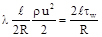,

where we find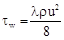(5)

We substitute (5) into equation (3) and obtain the following expression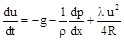(6)

We calculate the pressure gradient of the following conditions: pressure decreases linearly from the boost pressure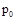over the free surface of the fuel to the pressure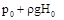. The pressure gradient is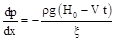(7)

where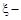coordinate of free fuel surface in the measuring channel.

In view of the formula (7) the equation of motion (6) takes the form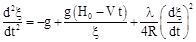(8)

we are adding initial conditions and obtain the Cauchy problem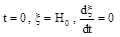(9)

4. Results

Performed numerical solution of the problem: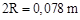,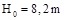,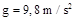,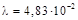,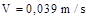. The solution of the boundary value problem is given numerically in package Mathcad applications. Results of the solution are shown in the graphs (Figure 2 - Figure 4)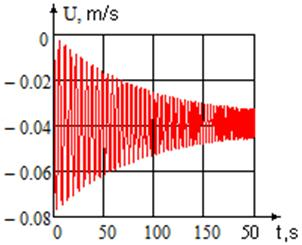Figure 2. Changing the average fluid velocity in the channel.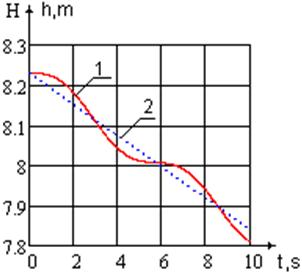Figure 3. Level of liquid: 1- in the channel, 2- in the tank.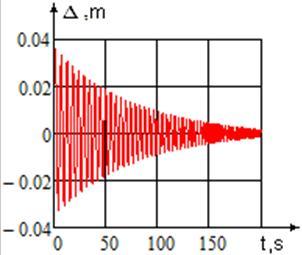Figure 4. Error in the measurement the liquid level in the range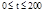s,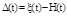.

5. Discussion

We can see (Figure 2), that the average velocity of the fluid in the channel has a decreasing amplitude oscillations. The liquid level in the cylindrical channel fluctuates relative to the liquid level in the fuel tank (Figure 3). The cause of the oscillation is the inertia of the liquid column in the channel. At the initial time the liquid has a very great acceleration, that causes a great inertia force of the. Consequently, the force of inertia is the cause fluctuations of the liquid in the channel. Summand in the equation of motion, which determines the oscillation process, is a pressure gradient.

Fluctuations have a variable period. Mathematical experiment shows that the period of oscillation decreases with time: in the time interval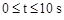period of oscillations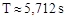; in the time interval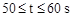period of oscillation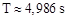. On the flow characteristics significantly affected by acceleration, so for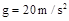andin the range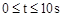the oscillation amplitude is reduced to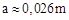, and the period is reduced to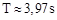. In comparison with the acceleration channel resistance effect was insignificant, so for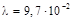and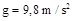in the rangeoscillation amplitude is practically unchanged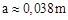and the period of oscillations is reduced to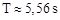.

The graph (Figure 4) shows the error in measuring the liquid level versus time. We can see that the magnitude of the error is a periodic function, in which the amplitude and period of oscillations decreases with time. The maximum error in determining the level of fuel in the tank is observed in the beginning of the flight of a rocket and reaches the value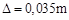. Error in measuring the liquid level is not regular, making it difficult to adjustment errors in software.

Note that the sensor may operate as in the positive and as in the negative deviation from the actual position of the fuel level in the tank. In this case, the error is doubled. Given the size of the diameter of the rocket, we are talking about hundreds of fuel kilograms.

6. Conclusion

The results suggest that the level sensors may provide false information, depending on the site of attachment. The error is not systematic. Experimental research is time-consuming and expensive. Therefore, the mathematical experiment is the most efficient method of solving the problem. The task is to signal from the sensor corresponded to the actual level of the liquid. For this purpose, the sensors must be installed at the points of intersection of the functions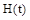and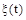.

It should be noted that the mathematical model of fluid flow in the vertical channel of management systems do not take into account fluctuations of liquid in the fuel tank rocket and some rocket design features. Such a study will be done in the next article.

References

1. Slezkin N.A. Dynamics of viscous incompressible fluid / A.T. Slezkin. M.: Gostekhizdat, 1955. 520 pp.(in Russ.)
2. Loytsyansky L.G. Fluid Mechanics. M. Science, 1970. 904 pp.(in Russ.)
3. Popov D.N. Unsteady hydromechanical processes. M.G. Machinery, 1982. 240 pp.(in Russ.)
4. Babe G.D., Bondarev E.A., Voevodin A.F., Kanibolotsky M.A. Identification of hydraulic models. Novosibirsk. Science. 1980, 160 pp.(in Russ.)
5. Ozawa, K., Shimada, T. Flight performance simulations of vertical launched sounding rockets using altering-intensity swirling-oxidizer-flow-type hybrid motors. 51st AIAA/SAE/ASEE Joint Propulsion Conference. 2015. Orlando, United States. 2015, 21 p.
6. Liu, Z., Feng, Q. Numerical analysis of gas pulsation attenuation characteristics of a perforated tube in a reciprocating compressor piping system. Proceedings of the Institution of Mechanical Engineers, Part A: Journal of Power and Energy. V. 230, Issue 1, 1 February 2016, pp. 99-111.
7. Majd, A., Ahmadi, A. Keramat, A. Investigation of non-Newtonian fluid effects during transient flows in a pipeline. Strojniski Vestnik/Journal of Mechanical Engineering. V. 62, Issue 2, 2016, pp. 105-115.
8. Wang, C., Yang, J. D. Water hammer simulation using explicit-implicit coupling methods. Journal of Hydraulic Engineering. V. 141, Issue 4. Article number 04014086.
9. Avramenko, A. A., Tyrinov, A. I., Shevchuk, I. V. An analytical and numerical study on the start-up flow of slightly rarefied gases in a parallel-plate channel and a pipe. Physics of Fluids. V. 27, Issue 4, 2015, Article number 1.4916621.
10. Noorani, A., Schlatter, P. Evidence of sublaminar drag naturally occurring in a curved pipe. Physics of Fluids V. 27, Issue 3. 2015, Article number 1.4913850.
11. Jafari Behbahani, T., Dahaghin, A., Behbahani, Z. J. Modeling of Flow of Crude Oil in a Circular Pipe Driven by Periodic Pressure Variations. Energy Sources, Part A: Recovery, Utilization and Environmental Effects. V. 37, Issue 13, 2015, pp. 1406-1414.
12. Simão, M., Mora-Rodriguez, J., Ramos, H. M. Mechanical interaction in pressurized pipe systems: Experiments and numerical models. Water (Switzerland). V.7, Issue 11, 2015, pp. 6321-6350.
13. Korade, I , Virag, Z., Šavar, M. Numerical simulation of one-dimensional flow in elastic and viscoelastic branching tube. 11th World Congress on Computational Mechanics, WCCM 5th European Conference on Computational Mechanics, ECCM 2014 and 6th European Conference on Computational Fluid Dynamics, ECFD 2014. 2014, pp. 7124-7131.

 Contents 1. 2. 3. 4. 5. 6.
Article ToolsAbstractPDF(649K)